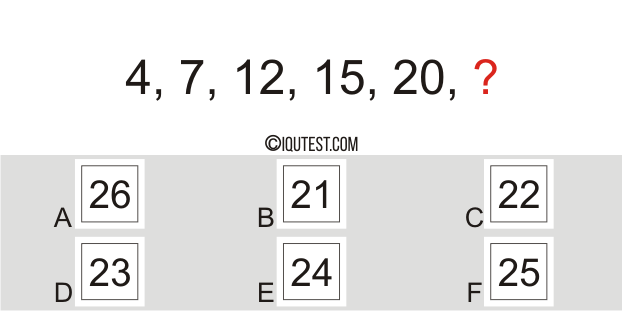# IQ Test Numerical [Number Series]

This test is a series of tests used to measure the ability to understand the pattern of numerical calculations using systematical series of numbers.

Find one of the right answers to complete the order of numbers on each question. See the example of number series test below.

Example Question:
Find The Missing Number?Explanations:

Numerical Test with Number Series
• 20 questions
• Numerical, Logical, Mathematic
• Time: 20 minutes

## More Challenging Tests